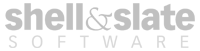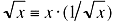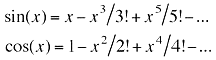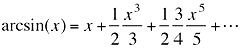### Computer Math 101

back

Ben Weiss

August 2001

Do not use or distribute without express permission of the author.

Email: ben@shellandslate.com

Over the course of years of programming, I’ve developed a substantial toolkit of math tricks to improve and optimize my code, primarily for the Macintosh platform. These tricks range from the simple to the very complex, but I have found them to be extremely useful, and I would like to present a few of them here.

First, a few typedefs to get into the spirit of things. All of the code below will use these conventions:

typedef unsigned char flag

typedef float         real32

typedef double       real64

typedef unsigned long uint32

typedef long          int32

1. Infinities

On the Mac, any reference to the constants HUGE_VAL or INFINITY (defined in fp.h) will actually generate a function call(!!) when used in actual code. However, if you try to define a constant double to be out of range, e.g.

real64 inf64 = 1.0e+500;

the compiler will complain that the constant is out of range, and won’t compile.

On the other hand, the compiler will allow single-precision values to be assigned double-precision constants, e.g.

real32 inf32 = 1.0e+40;

which will automatically overflow to positive infinity. Furthermore, a single-precision infinity converted to double precision will stay infinity, so we can define the following functions:

static inline real32 inf() { return 1.0e+40; }

static inline real32 minf() { return –1.0e+40; }

Any call to inf(), whether assigned to a single or double precision variable, will yield the correct result.

2. Square Roots

It’s ironic that the standard math libraries all define a square root function, but never a square root inverse. Normalizing a vector invariably requires dividing by the square root of the magnitude, which is equivalent to multiplying by the inverse sqrt. Since a multiply is much faster than a divide, wouldn’t an inverse sqrt be preferable to have? In any case, the square root can easily be reconstructed from the inverse square root by the following identity:With that in mind, here is some fast code to compute the inverse square root, using a Newton’s Method approximation technique. On the Mac platform, there is an intrinsic function ‘__frsqrte’ that computes an approximate inverse square root, which we can use as a seed value for the Newton iteration; on other platforms this approximation can be computed with the simple function below. As with the other methods I present here, a faster single-precision implementation is included for completeness.

#if !MacOS_

// ============================================================

// Inverse sqrt approximation, accurate to ~4.4 bits

// ============================================================

static inline real32 __frsqrte(real32 x, uint32 magic = 0x5F3997BB) {

uint32 q = *(uint32*)&x;

*(uint32*)&x = magic - ((q >> 1) & 0x3FFFFFFF);

return x;

}

#endif

// ============================================================

// Inverse sqrt, single-precision

// ============================================================

static inline real32 sqrtinv__(real32 fp0) {

real32 _min = 1.0e-38;

real32 _1p5 = 1.5;

real32 fp1,fp2,fp3;

fp2 = __frsqrte(fp0);

fp1 = _1p5 * fp0 - fp0;

fp3 = fp2 * fp2;       if(fp0 < _min)           goto uflow;

fp3 = _1p5 - fp1 * fp3;

fp2 = fp2 * fp3;

fp3 = fp2 * fp2;

fp3 = _1p5 - fp1 * fp3;

fp2 = fp2 * fp3;

fp3 = fp2 * fp2;

fp3 = _1p5 - fp1 * fp3;

return fp2 * fp3;

uflow:

return fp0 > 0 ? fp2 ::inf();

}

// ============================================================

// Inverse sqrt, double-precision

// ============================================================

static inline real64 sqrtinv__(real64 fp0) {

real64 _min = 1.0e-307;

real32 _1p5 = 1.5;

real64 fp1,fp2,fp3;

fp2 = __frsqrte(fp0);

fp1 = _1p5 * fp0 - fp0;

fp3 = fp2 * fp2;       if(fp0 < _min)           goto uflow;

fp3 = _1p5 - fp1 * fp3;

fp2 = fp2 * fp3;

fp3 = fp2 * fp2;

fp3 = _1p5 - fp1 * fp3;

fp2 = fp2 * fp3;

fp3 = fp2 * fp2;

fp3 = _1p5 - fp1 * fp3;

fp2 = fp2 * fp3;

fp3 = fp2 * fp2;

fp3 = _1p5 - fp1 * fp3;

return fp2 * fp3;

uflow:

return fp0 > 0 ? fp2 : real64(::inf());

}

3. Sines and Cosines

Another glaring oversight of the Mac toolkit is that there’s no function that returns the sine and cosine of a single value at once. Since most methods of computing sine and cosine actually end up computing them both, it seems like an awful waste of cycles to have to call first sin(), then cos().

In practice, calculating sincos(theta) requires the following steps:

1)    Reduce theta to the interval [0..2π];

2)    Reduce theta to the quadrant [0..π/2];

3)    Look up exact sincos values for some angle ‘phi’ close to theta;

4)    Compute the exact sincos values for ‘theta – phi’;

5)    Multiply sincos(phi) and sincos(theta – phi) to obtain sincos(theta);

6)    Expand from [0..π/2] back to [0..2π].

Each of these steps can benefit from clever computational tricks to increase efficiency. Here is a breakdown:

(1) Reduce theta to the interval [0..2π]

Rather than call ::fmod(theta, 2π), which would be excruciatingly slow, it makes more sense to divide theta by 2π (i.e., multiply by 1/2π), then reduce it to the interval [0..1]. The single-precision and double-precision methods use different tricks to accomplish this efficiently.

In single-precision, we make use of a large real constant which, when added to a single-precision value, places the fractional component into the lowest 32 bits of the 64-bit sum. This value happens to be 1.5 * 2^20, also representable in single-precision, though the addition must be performed in double-precision. Since single-precision values have only 23 bits of fractional information, the 32 bits we obtain are sufficient to adequately represent the modular angle between 0 and 1.

In double-precision, we can use a similar trick to the one the compiler uses to translate integer to floating-point. We can find a huge constant such that, when added to a double-precision angle, yields only enough bits of precision to represent the integer part of the angle. Subtracting the huge constant again yields the angle rounded to the nearest integer, and we can subtract this from the original angle to yield the fractional part, with full precision. If the final fraction is > 0 we add 1, otherwise we add 2, and the final value is guaranteed to be between 1 and 2 (except in pathological cases such as infinities or NaN’s).

(2) Reduce theta to the quadrant [0..π/2]

We do this because it simplifies the computation, ensuring that both sin and cos will be positive. In single-precision, the top two bits of the 32-bit fraction indicate the quadrant, and the other 30 bits fill the range [0..π/2]. In double-precision, similarly, the first two bits of the mantissa (of the angle between 1 and 2) specify the quadrant, and the remainder scales to [0..π/2].

(3) Look up exact sincos values for some angle ‘phi’ close to theta

At this point we have the angle essentially in an integer representation, with the top two bits specifying the quadrant. The following 10 bits (8 bits for single-precision) can be used to index into an array of precalculated values for sin(x), making use of the fact that sin(x) == cos(π / 2 – x). This method also protects us against reading out of bounds for pathological input values, that might not resolve to an angle between 1 and 2, as shown in step (1). The index bits serve the additional purpose of specifying the exact angle ‘phi’, which we simply subtract from theta to give us the delta value we still need to calculate.

(4) Compute the exact sincos values for ‘theta – phi’

Since ‘theta – phi’ is very small at this point, the Taylor expansion series for sin(x) and cos(x) converges very rapidly:(5) Multiply sincos(phi) and sincos(theta – phi) to give sincos(theta)

In this phase, we simply treat sincos(phi) and sincos(theta – phi) as complex numbers, where multiplication has the serindipitous effect of adding the angles of the multiplicands. The result will give us the exact sincos(theta), on the interval [0..π/2].

(6) Expand from [0..π/2] back to [0..2π].

Using the afforementioned two most significant bits of the angle, we shuffle the quadrants to yield the final sincos(theta), on [0..2π]. Done!

Here is the actual code, for single and double-precision values, starting with the initialization code required to precompute the arrays (using the full-precision system sin() and cos()):

static flag sincosinited = false;

static real32 sinbuf_;

static real64 dsinbuf_;

#define _2pi (2.0 * 3.1415926535897932384626434)

#define _2pif real32(_2pi)

#if LittleEndian_

enum { iexp_ = 1, iman_ = 0 }

#else

enum { iexp_ = 0, iman_ = 1 }

#endif

// =====================================================================

// init sincos buffers, single and double precision

// =====================================================================

static void initsincos_() {

if (sincosinited)                 return;

real64 angle = _2pi / (4 * 256);

uint32 ang;

for (ang = 0; ang <= 128; ang++) {

sinbuf_[ ang] = ::sin(angle * ang) * 2;

sinbuf_[256 - ang] = ::cos(angle * ang) * 2;

}

angle = _2pi / (4 * 1024);

for (ang = 0; ang <= 512; ang++) {

dsinbuf_[ ang] = ::sin(angle * ang);

dsinbuf_[1024 - ang] = ::cos(angle * ang);

}

sincosinited = true;

}

// =====================================================================

// sincos, single-precision (initsincos_() must be called in advance)

// =====================================================================

static void sincos_(real32 theta, real32& s, real32& c) {

const real32 _1p5m       = 1572864.0;

const real64 _2piinv     = 1.0 / _2pi;

const real32 _pi512inv   = _2pi / 1024.0;

real64 thb = theta * _2piinv + _1p5m;

// ____ read 32 bit integer angle

uint32 thi = ((uint32*)(&thb))[iman_];

// ____ first 8 bits of argument, in [0..π/2]

uint32 th = (thi >> 20) & 0x03FC;

pointer sbuf = sinbuf_;

// ____ read approximate sincos * 2

real32 st = *(real32*)(address(sbuf) + (  th));

real32 ct = *(real32*)(address(sbuf) + (1024 - th));

uint32 tf = (thi & 0x003FFFFF) | 0x3F800000;

real32 sd = (*(real32*)(&tf)) * _pi512inv - _pi512inv;

real32 cd = 0.5f - sd * sd;

real32 sv = sd * ct + cd * st;

real32 cv = cd * ct - sd * st;

if (int32(thi) >= 0) {

if (thi & 0x40000000)    { s = cv; c = -sv; }

else                     { s = sv; c = cv; }

}

else {

if (thi & 0x40000000)    { s = -cv; c = sv; }

else                     { s = -sv; c = -cv; }

}

}

// =====================================================================

// sincos, double-precision (initsincos_() must be called in advance)

// =====================================================================

static void sincos_(real64 theta, real64& s, real64& c) {

real32 _2e52p5 = 1048576.0 * 1048576.0 * 4096.0 * 1.5;

real64 _2piinv = 1.0 / _2pi;

real64 thb = (_2e52p5 + _2piinv * theta) - _2e52p5;

theta = (theta * _2piinv - thb);

if(theta > 0)    theta += 1;  // 1..2

else             theta += 2;  // 1..2

real64 buf;

*buf = theta;

uint32 thi = ((uint32*)(&buf))[iexp_];

uint32 th8 = thi >> 5 & 0x1FF8;

((uint32*)(&buf))[iexp_] = thi & 0xFFF000FF;

theta = *buf * _2pi - _2pi;  // [0 .. 2π / 4096]

real64 st = *(real64*)(address(dsinbuf_) + ( th8));

real64 ct = *(real64*)(address(dsinbuf_) + (8192 - th8));

// ____ calculate delta sincos explicitly

real64 theta2 = theta * theta;

real64 theta3 = theta2 * theta;

real64 theta4 = theta2 * theta2;

real64 sd = theta - theta3 * real64(1.0 / 6.0);

real64 cd = theta2 * 0.5f - theta4 * real64(1.0 / 24.0);

// ____ multiply to obtain final sincos

real64 sv = (st + sd * ct) - cd * st;

real64 cv = (ct - cd * ct) - sd * st;

// ____ expand to [0..2π]

if (thi & 0x00080000) {

if (thi & 0x00040000)    { s = -cv; c = sv; }

else                     { s = -sv; c = -cv; }

}

else {

if (thi & 0x00040000)    { s = cv; c = -sv; }

else                     { s = sv; c = cv; }

}

}

4. Arctangents

In a nutshell, the most useful application for the arctangent function is to convert between rectangular and polar coordinates. To be more specific, starting with the location (x, y), we want to find (theta, r) in polar coordinates. This calculation is usually performed as follows:

theta = ::atan2(y, x);

r = ::sqrt(x * x + y * y);

As with the sincos() case illustrated above, cycles are being thrown away here. The calculation of the arctangent automatically generates the square root as a by-product, so why not use it?

To this end, I’ve written the following methods:

real32 atan2r_(real32 y, real32 x, real32& r);

real64 atan2r_(real64 y, real64 x, real64& r);

which return the magnitude of the vector (x, y) in r, as well as the arctangent. Similarly to the sincos function, computing the atan can be reduced to the following steps:

1)    Normalize the vector (x, y), and reduce to the octant [0..π/4];

2)    Look up exact arcsin, cos values for some angle ‘phi’ near theta;

3)    Compute arcsin explicitly for ‘theta – phi’;

4)    Add the two arcsins to get arctan(theta);

5)    Expand back out to the interval [-π..π].

(1) Normalize the vector (x, y), and reduce to the octant [0..π/4]

This operation involves dividing (x, y) by the quantity sqrt(x^2 + y^2), which we can perform very efficiently (and without a floating-point divide!), using the sqrtinv_() method above. The octant reduction is done by direct comparison and branching, and ensures that 0 <= y <= x, which simplifies the remaining computation. The vector magnitude is also returned at this point.

(2) Look up exact arcsin, cos values for some angle ‘phi’ near theta

Since our vector (x, y) is now normalized, we know that y == sin(theta). So we can index into a table of values to find arcsin(phi), for some angle phi close to theta. Our index value gives us sin(phi), and we also look up cos(phi), which will aid us in our calculation.

(3) Compute arcsin explicitly for ‘theta – phi’

Since we already have (x, y) == sincos(theta), and we’ve looked up sincos(phi), we can multiply them together (actually, sincos(theta) * sincos(-phi)), to obtain sincos(theta – phi). From our new value sin(theta – phi), which is small, we can use the elegant Taylor expansion of the arcsin function:to efficiently compute arcsin(theta – phi).

(4) Add the two arcsins to get arctan(theta)

This part seems almost magically simple, but mathematically it works out. (Trust me.)

(5) Expand back out to the interval [-π..π]

This step simply unwinds the reduction we performed in step (2), and yields the final arctangent value. Done!

To complete the suite of arctangent methods, it’s trivial to modify the following code to produce versions that return the inverse of the magnitude (useful for normalizing), no magnitude at all (simulating atan2), or atan(x) of one variable by observing that atan(x) == atan2(x, 1), on the interval [-π/2 .. π /2]. Here is the code, accurate to within 4 lsb’s in double precision:

static flag ataninited = false;

static real32 atanbuf_[257 * 2];

static real64 datanbuf_[513 * 2];

// ====================================================================

// arctan initialization

// =====================================================================

static void initatan_() {

if (ataninited)                   return;

uint32 ind;

for (ind = 0; ind <= 256; ind++) {

real64 v = ind / 256.0;

real64 asinv = ::asin(v);

atanbuf_[ind * 2    ] = ::cos(asinv);

atanbuf_[ind * 2 + 1] = asinv;

}

for (ind = 0; ind <= 512; ind++) {

real64 v = ind / 512.0;

real64 asinv = ::asin(v);

datanbuf_[ind * 2    ] = ::cos(asinv);

datanbuf_[ind * 2 + 1] = asinv;

}

ataninited = true;

}

// =====================================================================

// arctan, single-precision; returns theta and r

// =====================================================================

real32 atan2r_(real32 y_, real32 x_, real32& r_) {

Assert(ataninited);

real32 mag2 = x_ * x_ + y_ * y_;

if(!(mag2 > 0))  { goto zero; }   // degenerate case

real32 rinv = sqrtinv_(mag2);

uint32 flags = 0;

real32 x, y;

real32 ypbuf;

real32 yp = 32768;

if (y_ < 0 ) { flags |= 4; y_ = -y_; }

if (x_ < 0 ) { flags |= 2; x_ = -x_; }

if (y_ > x_) {

flags |= 1;

yp += x_ * rinv; x = rinv * y_; y = rinv * x_;

ypbuf = yp;

}

else {

yp += y_ * rinv; x = rinv * x_; y = rinv * y_;

ypbuf = yp;

}

r_ = rinv * mag2;

int32 ind = (((int32*)(ypbuf)) & 0x01FF) * 2 * sizeof(real32);

real32* asbuf = (real32*)(address(atanbuf_) + ind);

real32 sv = yp - 32768;

real32 cv = asbuf;

real32 asv = asbuf;

sv = y * cv - x * sv;    // delta sin value

// ____ compute arcsin directly

real32 asvd = 6 + sv * sv;   sv *= real32(1.0 / 6.0);

real32 th = asv + asvd * sv;

if (flags & 1) { th = _2pif / 4 - th; }

if (flags & 2) { th = _2pif / 2 - th; }

if (flags & 4) { return -th; }

return th;

zero:

r_ = 0; return 0;

}

// =====================================================================

// arctan, double-precision; returns theta and r

// =====================================================================

real64 atan2r_(real64 y_, real64 x_, real64& r_) {

Assert(ataninited);

const real32 _0 = 0.0;

real64 mag2 = x_ * x_ + y_ * y_;

if(!(mag2 > _0)) { goto zero; }   // degenerate case

real64 rinv = sqrtinv_(mag2);

uint32 flags = 0;

real64 x, y;

real64 ypbuf;

real64 _2p43 = 65536.0 * 65536.0 * 2048.0;

real64 yp = _2p43;

if (y_ < _0) { flags |= 4; y_ = -y_; }

if (x_ < _0) { flags |= 2; x_ = -x_; }

if (y_ > x_) {

flags |= 1;

yp += x_ * rinv; x = rinv * y_; y = rinv * x_;

ypbuf = yp;

}

else {

yp += y_ * rinv; x = rinv * x_; y = rinv * y_;

ypbuf = yp;

}

r_ = rinv * mag2;

int32 ind = (((int32*)(ypbuf))[iman_] & 0x03FF) * 16;

real64* dasbuf = (real64*)(address(datanbuf_) + ind);

real64 sv = yp - _2p43; // index fraction

real64 cv = dasbuf;

real64 asv = dasbuf;

sv = y * cv - x * sv;    // delta sin value

// ____ compute arcsin directly

real64 asvd = 6 + sv * sv;   sv *= real64(1.0 / 6.0);

real64 th = asv + asvd * sv;

if (flags & 1) { th = _2pi / 4 - th; }

if (flags & 2) { th = _2pi / 2 - th; }

if (flags & 4) { th = -th; }

return th;

zero:

r_ = _0; return _0;

}

Benchmark Results (Mac G4/500)

Sqrt:          1000000 samples from 0.5 to 1.5

Sincos:      2000000 samples from –π to π

Atan2:        2000000 samples from –π to π

Note that since we have access to the source code, these methods can be inlined for even greater speed gains. (For fair comparison with system functions, these benchmarks reflect non-inlined speeds. The sqrtinv_ methods are only declared inline so they will be inlined in the atan2r_ methods.)

Error is expressed in lsb’s (least significant bits) in the target format.

 Operation Cycles RMS Error (LSB bits) Max Error (LSB bits) sqrtinv_ (single) 47 0.32 1 sqrtinv_ (double) 54 0.46 2 OS: sqrt 96 OS: 1.0f / real32(sqrt) 118 OS: 1.0 / sqrt 131 sincos_ (single) 50 0.23 1 sincos_ (double) 71 1.65 6.5 OS: sin + cos 176 atan2r_ (single) 82 0.58 2.5 atan2r_ (double) 102 0.55 4 OS: atan2 334 OS: atan2 + sqrt 439

Shell & Slate Software Corp. accepts no liability for any numerical catastrophes, or other misadventures, resulting from unauthorized use of this code.